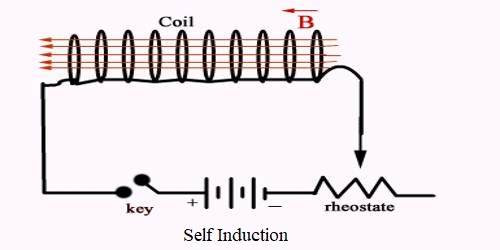Physics

# Coefficient of Self-inductanceCoefficient of self-induction or self-inductance

When current flows through a closed coil, then that current creates a magnetic field and the coil becomes associated with that field. So, if there is a change of current in the coil, associated magnetic field lines also change, and induced electromotive force is produced in the coil which opposes the very change of current. It is called self-induction. It is the induction of an electromotive force in a circuit when the current in that circuit is varied. The coefficient of self-induction is the ratio of the electromotive force produced in a  circuit by self-induction to the rate of change of current producing it, expressed in henries.

From the experimental results, it is seen that the magnetic flux passing through a closed loop is proportional to the current through that coil.

Let the magnetic flux enclosed be φ due to the flow of current ‘i’ in a coil.

So we get,

φ ∞ i,

or, φ = Li … … … (1)

Here L is a constant; it is called coefficient of self-induction or self-inductance. It depends on the geometrical factor of the coil and the permeability of the medium.

Now, let us define L in language.

Suppose, i = 1 (unit)

From equation (1) we get, φ = L

So, if one unit of current flows in a coil then the amount of magnetic flux contained in it is called the coefficient of sell induction or self-inductance of that coil.

E = dφ/dt

so, E = – d/dt (Li) = – L (di/dt) … … … (2)

Negative sign indicates that the induced electromotive force always opposes the change of current.

From equation (2) we can define L.

E = L (di/dt) [ignoring the negative sign]

or, L = E / (di/dt)

Now, if = (di/dt) = 1 then L = E

So, if the current in a coil changes by one unit per second, then the electromotive force induced in that coil is called the coefficient of self induction of that coil.

Unit of self-inductance: S.I. unit of self-inductance is Henry.

1 Henry: If due to the change of one ampere current per second in a coil, one volt of electromotive force is induced in that coil, then the self-inductance of the coil is 1 Henry.